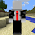## Back to Basics Challenge: Week 1 - The Fibonacci SequenceSometimes, as programmers, we get set in our ways. We tend to get lazy, and as a result, our code gets cluttered and unmanageable. Here’s a great example of a good developer that had to clean up their code. http://playrust.com/friday-devblog/

What I propose is to get back to CS 101 basics with a small twist. Over the next few weeks, I will be posting a series of programming challenges. Here are the rules:
1. Use any programming language that you want.
2. Perform the task as instructed in AS FEW lines of code as possible.
3. Brackets, comments, whitespace and display code don’t count toward line count.
4. Minification will hurt you.

## The Fibonacci Sequence

Anyone who has been in a programming class knows of this algorithm. Each number in the sequence is the sum of the previous two numbers. E.g. 1,1,2,3,5,8,13,21…

What you have to do for this first challenge is generate an array that contains the first 20 iterations of the Fibonacci Sequence.

Here is my submission in PHP: 4 lines according to the rules.

<?php
\$seq = array();
\$last = 0;
for (\$i = 0; \$i <= 19; \$i++) {
\$i == 0 || \$i == 1 ? \$last = \$seq[] = 1 : \$last = \$seq[] = \$seq[\$i - 1] + \$seq[\$i - 2];
}
print_r(\$seq);

Good Luck!

1.Here is my 4 line PHP attempt:

\$stack = array(\$last=1, \$current=1);
while(count(\$stack) < 20){
array_push(\$stack, (\$current += \$last));
\$last = \$current - \$last;
}print_r(\$stack);

2.for (\$x=1; \$x<=20; \$x++) {
\$fibArray[\$x] = \$x <= 1 ? \$x : \$fibArray[\$x-2] + \$fibArray[\$x-1];
}
print_r(\$fibArray);

1.3.Does this count as 1?
for (\$x=1, \$fibArray = array(0,1); \$x<=20; \$fibArray[\$x] = (\$x <= 1 ? \$x : \$fibArray[\$x-2] + \$fibArray[\$x-1]), \$x++){}
print_r(\$fibArray);

1.This comment has been removed by the author.

2.it prints one to many times ;)

4.Perbaikan sistem pada sebuah game sangatlah penting, karena Maintenance Rutin Poker Online akan mempengaruhi baik dan buruk nya sistem permainan tersebut. Contoh saja pada permainan poker online di s1288poker, selalu memiliki jadwal rutin untuk maintenance disetiap minggu nya (Baca Selengkapnya...)